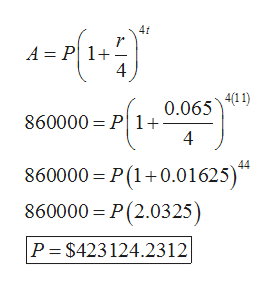# A corporation creates a sinking fund in order to have \$860,000 to replace some machinery in 11 years. How much should be placed in this account at the end of each quarterif the annual interest rate is 6.5% compounded quarterly? (Round your answers to the nearest cent.)\$ How much interest would they earn over the life of the account?\$ Determine the value of the fund after 2, 4, and 6 years.2 years \$4 years \$6 years \$How much interest was earned during the third quarter of the 4th year?\$

Question
196 views

A corporation creates a sinking fund in order to have \$860,000 to replace some machinery in 11 years. How much should be placed in this account at the end of each quarterif the annual interest rate is 6.5% compounded quarterly? (Round your answers to the nearest cent.)
\$

How much interest would they earn over the life of the account?
\$

Determine the value of the fund after 2, 4, and 6 years.

 2 years \$ 4 years \$ 6 years \$

How much interest was earned during the third quarter of the 4th year?
\$

check_circle

Step 1

Hello. Since your question has multiple sub-parts, we will solve first three sub-parts for you. If you want remaining sub-parts to be solved, then please resubmit the whole question and specify those sub-parts you want us to solve.

Now come to the question:

According to the given question:

Amount (A) =\$860,000

Time (t) =11 years

Rate of interest(r) =6.5%

It is needed to calculate the amount to be placed at the end of each quarter and the interest earned over the life of the account.

Step 2

Formula used to calculate amount that is compounded quarterly is:

Step 3

Now substitute the value...help_outlineImage Transcriptionclose4f A P 1+ 4/11) 0.065 860000 P 1+ - 4 860000 P(1+0.01625) 860000 P(2.0325) P \$423124.2312 fullscreen

### Want to see the full answer?

See Solution

#### Want to see this answer and more?

Solutions are written by subject experts who are available 24/7. Questions are typically answered within 1 hour.*

See Solution
*Response times may vary by subject and question.
Tagged in

### Math